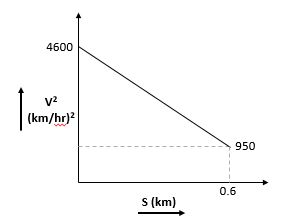# Graph between the square of velocity (v) of a particle and the distance (s) moved is shown in figure. The acceleration of the particle in kilometers per hour square is:Option 1) 2250 Option 2) 3084 Option 3) -2250 Option 4) -3084

As learnt in

3rd equation or Velocity –displacement equation -Final VelocityDisplacementInitial velocityacceleration

-

We use V2 = u2 + 2as

V2 - u2 = 2as

After solving we get acceleration of particle -3084

Option 1)

2250

Option 2)

3084

Option 3)

-2250